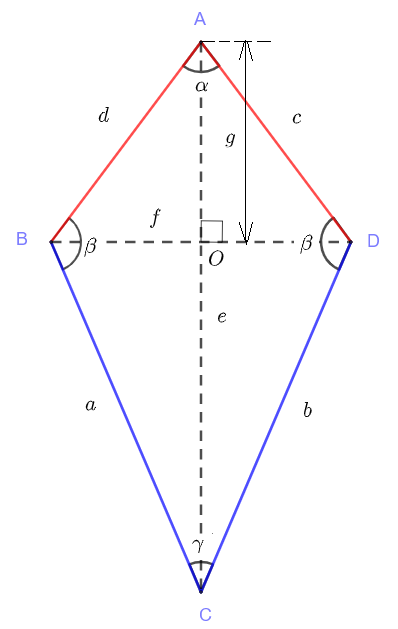# Kite Calculator

An online calculator to calculate the sides, area, perimeter and angles in a kite given its diagonals and distance $AO$.We define the length of segments $AC$, $BD$ and $AO$ using small letters as follows: $AC = e$, $BD = f$ and $AO = g$.
The
kite formulas are first given.
Area $A$ of Kite: $\displaystyle A = \dfrac{f \cdot e}{2}$
Sides $a$ and $b$: $\displaystyle a = b = \sqrt{ \left(\dfrac{f}{2}\right)^2 + (e-g)^2}$
Sides $c$ and $d$: $\displaystyle d = c = \sqrt{ \left(\dfrac{f}{2}\right)^2 + g^2}$
Perimeter: $\displaystyle p = 2 a + 2 d$
Angle $\alpha$: $\displaystyle \alpha = 2 \arctan (\dfrac{f}{2g})$
Angle$\gamma$ : $\displaystyle \gamma = 2 \arctan \left(\dfrac{f}{2(e-g)}\right)$
Angle $\beta$: $\displaystyle \beta = 180 - \dfrac{\gamma}{2} - \dfrac{\alpha}{2}$

## Use of the Kite Calculator

Enter the lengths $e$ of $AC$, the length $f$ of $BD$ and the length $g$ of $AO$ as positive real numbers such that $g \lt e$.

 Axis Length $AC$: $\quad e$ = 0.8 Diagonal Length $BD$: $\quad f$ = 0.4 $AO$: $\quad g$ = 0.2 Number of Decimls = 3